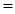Chapter 5, Problem 110SCQ

Chapter
Section
Textbook Problem

Peanuts and peanut oil are organic materials and bum in air. How many burning peanuts does it take to provide the energy to boil a cup of water (250 mL of water)? To solve this problem, we assume each peanut, with an average mass of 0.73 g, is 49% peanut oil and 21% starch; the remainder is noncombustible We further assume peanut oil is palmitic acid, C16H32O2, with an enthalpy of formation of −848.4 kJ/mol. Starch is a long chain of C6H10O5 units, each unit having an enthalpy of formation of −960 kJ.

Interpretation Introduction

Interpretation:

To identify how many burning peanuts are required to boil 250mL of water

Concept Introduction:

Heat energy required to raise the temperature of 1g of substance by 1K.Energy gained or lost can be calculated using the below equation.

q=C×m×ΔT

Where, q= energy gained or lost for a given mass of substance (m), C =specific heat capacity,ΔT= change in temperature.

The enthalpy of combustion can be calculated as

ΔcH0ΣnΔfH0(reactants)-ΣnΔfH0(products)

Explanation

Given 49% of the peanut is palmitic acid.

0.73g×49100 = 0.3577g of palmitic acid.

Given 21% of the starch is palmitic acid.

0.73g×21100=0.153g of starch

Molecular weight of starch=162g/mol

So we have 0.15162 = 0.000925 moles of starch.

Enthalpy of combustion of palmitic acid.is calculated as,

C16H32O2+23O216O2+16H2O

Substitute in ΔcH0ΣnΔfH0(reactants)-ΣnΔfH0(products) as

ΔcH0=(848.4+0)-(16× -393.5+16×241.8)=-9315.6kJ/mol

Enthalpy of combustion of starch.is calculated as

C6H10O5+6O26O2+5H2O

ΔcH0=(-960+0)-(6× -393.5+-241.8)=-2610kJ/mol

Since we have 0.000925 moles of starch = 0.000925 moles × -2610kJ/mol

=-2

Still sussing out bartleby?

Check out a sample textbook solution.

See a sample solution

The Solution to Your Study Problems

Bartleby provides explanations to thousands of textbook problems written by our experts, many with advanced degrees!

Get Started

Find more solutions based on key concepts